# Ground state and mountain pass solutions for discrete $p\left(\cdot \right)$-Laplacian

## Abstract

In this paper we study the existence of solutions for discrete $p\left(\cdot \right)$-Laplacian equations subjected to a potential type boundary condition. Our approach relies on Szulkin’s critical point theory and enables us to obtain the existence of ground state as well as mountain pass type solutions.

MSC: 39A12, 39A70, 49J40, 65Q10.

## 1 Introduction

Let T be a positive integer, $p:\left[0,T\right]\to \left(1,\mathrm{\infty }\right)$ and ${h}_{p\left(k\right)}:\mathbb{R}\to \mathbb{R}$ be defined by ${h}_{p\left(k\right)}\left(x\right)={|x|}^{p\left(k\right)-2}x$ for all $x\in \mathbb{R}$ and $k\in \left[0,T\right]$. Here and below, for $a,b\in \mathbb{N}$ with $a, we use the notation $\left[a,b\right]:=\left\{a,a+1,\dots ,b\right\}$.

This paper is concerned with the existence of solutions for equations of the type

$-{\mathrm{\Delta }}_{p\left(k-1\right)}x\left(k-1\right)=f\left(k,x\left(k\right)\right),\phantom{\rule{1em}{0ex}}\left(\mathrm{\forall }\right)k\in \left[1,T\right]$
(1.1)

subjected to the potential boundary condition

$\left({h}_{p\left(0\right)}\left(\mathrm{\Delta }x\left(0\right)\right),-{h}_{p\left(T\right)}\left(\mathrm{\Delta }x\left(T\right)\right)\right)\in \partial j\left(x\left(0\right),x\left(T+1\right)\right),$
(1.2)

where $\mathrm{\Delta }x\left(k\right)=x\left(k+1\right)-x\left(k\right)$ is the forward difference operator and ${\mathrm{\Delta }}_{p\left(\cdot \right)}$ stands for the discrete $p\left(\cdot \right)$-Laplacian operator, that is,

${\mathrm{\Delta }}_{p\left(k-1\right)}x\left(k-1\right):=\mathrm{\Delta }\left({h}_{p\left(k-1\right)}\left(\mathrm{\Delta }x\left(k-1\right)\right)\right)={h}_{p\left(k\right)}\left(\mathrm{\Delta }x\left(k\right)\right)-{h}_{p\left(k-1\right)}\left(\mathrm{\Delta }x\left(k-1\right)\right).$

Here and hereafter, $f:\left[1,T\right]×\mathbb{R}\to \mathbb{R}$ is a continuous function, while $j:\mathbb{R}×\mathbb{R}\to \left(-\mathrm{\infty },+\mathrm{\infty }\right]$ is convex, proper (i.e., $D\left(j\right):=\left\{z\in \mathbb{R}×\mathbb{R}:j\left(z\right)<+\mathrm{\infty }\right\}\ne \mathrm{\varnothing }$), lower semicontinuous (in short, l.s.c.) and ∂j denotes the subdifferential of j. Recall, for $z\in \mathbb{R}×\mathbb{R}$, the set $\partial j\left(z\right)$ is defined by

$\partial j\left(z\right)=\left\{\zeta \in \mathbb{R}×\mathbb{R}:j\left(\xi \right)-j\left(z\right)\ge \left(\zeta |\xi -z\right),\left(\mathrm{\forall }\right)\xi \in \mathbb{R}×\mathbb{R}\right\},$
(1.3)

where $\left(\cdot |\cdot \right)$ stands for the usual inner product in $\mathbb{R}×\mathbb{R}$.

It should be noticed that the boundary condition (1.2) recovers the classical ones. For instance, denoting by ${I}_{K}$ the indicator function of a closed, nonempty and convex set $K\subset \mathbb{R}×\mathbb{R}$, the Dirichlet and Neumann boundary conditions are obtained by choosing $j={I}_{K}$ with $K=\left\{\left(0,0\right)\right\}$ and $K=\mathbb{R}×\mathbb{R}$, respectively. If p is T-periodic, taking $K=\left\{\left(x,x\right),x\in \mathbb{R}\right\}$ ($K=\left\{\left(x,-x\right),x\in \mathbb{R}\right\}$) and $j={I}_{K}$, we get the periodic (antiperiodic) conditions. For other choices of j yielding various boundary conditions, we refer the reader to Gasinski and Papageorgiou  and Jebelean .

The study of boundary value problems with a discrete p-Laplacian using variational approaches has captured attention in the last years. Most of the papers deal with classical boundary conditions such as Dirichlet (see, e.g., Agarwal et al. , Cabada et al. ), Neumann (Candito and D’Agui , Tian and Ge ) and periodic (He and Chen , Jebelean and Şerban ). Also, we note the recent paper of Mawhin  where variational techniques are employed to obtain the existence of periodic solutions for systems involving a general discrete ϕ-Laplacian operator.

Boundary value problems with the discrete $p\left(\cdot \right)$-Laplacian subjected to Dirichlet, Neumann or periodic boundary conditions were studied in recent time by Bereanu et al. , Galewski and Glab [11, 12], Guiro et al. , Koné and Ouaro , Mashiyev et al. , Mihăilescu et al. [16, 17].

Here, we use a variational approach to obtain ground state and mountain pass solutions for problem (1.1), (1.2). In this view, we employ some ideas originated in Jebelean and Moroşanu  (also see Jebelean ) combined with specific technicalities due to the discrete and anisotropic character of the problem. The main existence results are Theorem 3.1 and Theorem 4.2. These recover and generalize the similar ones for p= constant obtained in .

The rest of the paper is organized as follows. The functional framework and the variational approach of problem (1.1), (1.2) are presented in Section 2. In Section 3, we obtain the existence of ground state solutions, while Section 4 is devoted to the existence of mountain pass type solutions. An example of application is given in Section 5.

## 2 The functional framework

Our approach for the boundary value problem (1.1), (1.2) relies on the critical point theory developed by Szulkin . With this aim, we introduce the space

$X:=\left\{x:\left[0,T+1\right]\to \mathbb{R}\right\},$

which will be considered with the Luxemburg norm

${\parallel x\parallel }_{\eta ,p\left(\cdot \right)}=inf\left\{\nu >0:\sum _{k=1}^{T+1}\frac{1}{p\left(k-1\right)}{|\frac{\mathrm{\Delta }x\left(k-1\right)}{\nu }|}^{p\left(k-1\right)}+\eta \sum _{k=1}^{T}\frac{1}{p\left(k\right)}{|\frac{x\left(k\right)}{\nu }|}^{p\left(k\right)}\le 1\right\}$

for some $\eta >0$. Also, we shall make use of the usual sup-norm ${\parallel x\parallel }_{\mathrm{\infty }}={max}_{k\in \left[0,T+1\right]}|x\left(k\right)|$.

Next, let $\phi :X\to \mathbb{R}$ be defined by

$\phi \left(x\right)=\sum _{k=1}^{T+1}\frac{1}{p\left(k-1\right)}{|\mathrm{\Delta }x\left(k-1\right)|}^{p\left(k-1\right)},\phantom{\rule{1em}{0ex}}\left(\mathrm{\forall }\right)x\in X.$
(2.1)

Standard arguments show that φ is convex, of class ${C}^{1}$. Using the summation by parts formula (see, e.g., [8, 19]), one obtains that its derivative is given by

$〈{\phi }^{\prime }\left(x\right),y〉=\sum _{k=1}^{T+1}{h}_{p\left(k-1\right)}\left(\mathrm{\Delta }x\left(k-1\right)\right)\mathrm{\Delta }y\left(k-1\right),\phantom{\rule{1em}{0ex}}\left(\mathrm{\forall }\right)x,y\in X.$
(2.2)

By means of j, we introduce the functional $J:X\to \left(-\mathrm{\infty },+\mathrm{\infty }\right]$ given by

$J\left(x\right)=j\left(x\left(0\right),x\left(T+1\right)\right),\phantom{\rule{1em}{0ex}}\left(\mathrm{\forall }\right)x\in X.$
(2.3)

Note that, as j is proper, convex and l.s.c., the same properties hold true for J. Then setting

$\psi =\phi +J,$
(2.4)

it is clear that ψ is proper, convex and l.s.c. on X.

Further, we define $F:\left[1,T\right]×\mathbb{R}\to \mathbb{R}$ by

$F\left(k,t\right)={\int }_{0}^{t}f\left(k,\tau \right)\phantom{\rule{0.2em}{0ex}}d\tau ,\phantom{\rule{1em}{0ex}}\left(\mathrm{\forall }\right)k\in \left[1,T\right],\left(\mathrm{\forall }\right)t\in \mathbb{R}$

and

$\mathrm{\Phi }\left(x\right)=-\sum _{k=1}^{T}F\left(k,x\left(k\right)\right),\phantom{\rule{1em}{0ex}}\left(\mathrm{\forall }\right)x\in X.$
(2.5)

It is a simple matter to check that $\mathrm{\Phi }\in {C}^{1}\left(X,\mathbb{R}\right)$ and

$〈{\mathrm{\Phi }}^{\prime }\left(x\right),y〉=-\sum _{k=1}^{T}f\left(k,x\left(k\right)\right)y\left(k\right),\phantom{\rule{1em}{0ex}}\left(\mathrm{\forall }\right)x,y\in X.$
(2.6)

The energy functional associated to problem (1.1), (1.2) is

$\mathcal{I}=\mathrm{\Phi }+\psi$

with ψ in (2.4) and Φ given by (2.5).

Proposition 2.1 If $x\in X$ is a critical point of the functional $\mathcal{I}$ in the sense that

$〈{\mathrm{\Phi }}^{\prime }\left(x\right),y-x〉+\psi \left(y\right)-\psi \left(x\right)\ge 0,\phantom{\rule{1em}{0ex}}\left(\mathrm{\forall }\right)y\in X,$
(2.7)

then x is a solution of problem (1.1), (1.2).

Proof In (2.7), we take $y=x+sw$, $s>0$; then dividing by s and letting $s\to {0}^{+}$, we get

$〈{\mathrm{\Phi }}^{\prime }\left(x\right),w〉+〈{\phi }^{\prime }\left(x\right),w〉+{J}^{\prime }\left(x;w\right)\ge 0,\phantom{\rule{1em}{0ex}}\left(\mathrm{\forall }\right)w\in X,$

where ${J}^{\prime }\left(x;w\right)$ is the directional derivative of the convex function J at x in the direction of w. By virtue of (2.3), the above inequality becomes

$〈{\mathrm{\Phi }}^{\prime }\left(x\right),w〉+〈{\phi }^{\prime }\left(x\right),w〉+{j}^{\prime }\left(\left(x\left(0\right),x\left(T+1\right)\right);\left(w\left(0\right),w\left(T+1\right)\right)\right)\ge 0,\phantom{\rule{1em}{0ex}}\left(\mathrm{\forall }\right)w\in X.$

Using (2.2), (2.6) and the summation by parts formula, a straightforward computation shows that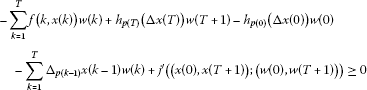(2.8)

for all $w\in X$. Thus, we infer

$\sum _{k=1}^{T}\left({\mathrm{\Delta }}_{p\left(k-1\right)}x\left(k-1\right)+f\left(k,x\left(k\right)\right)\right)w\left(k\right)=0$

for all $w\in X$ with $w\left(0\right)=w\left(T+1\right)=0$. This implies that

$-{\mathrm{\Delta }}_{p\left(k-1\right)}x\left(k-1\right)=f\left(k,x\left(k\right)\right),\phantom{\rule{1em}{0ex}}\left(\mathrm{\forall }\right)k\in \left[1,T\right].$
(2.9)

To prove that x satisfies condition (1.2), we multiply the equality (2.9) by $w\left(k\right)$. Then summing from 1 to T and using (2.8), one obtains

${j}^{\prime }\left(\left(x\left(0\right),x\left(T+1\right)\right);\left(w\left(0\right),w\left(T+1\right)\right)\right)\ge -{h}_{p\left(T\right)}\left(\mathrm{\Delta }x\left(T\right)\right)w\left(T+1\right)+{h}_{p\left(0\right)}\left(\mathrm{\Delta }x\left(0\right)\right)w\left(0\right)$

for all $w\in X$. Taking $w\in X$ with $w\left(0\right)=u$ and $w\left(T+1\right)=v$, where $u,v\in \mathbb{R}$ are arbitrarily chosen, we have

${j}^{\prime }\left(\left(x\left(0\right),x\left(T+1\right)\right);\left(u,v\right)\right)\ge {h}_{p\left(0\right)}\left(\mathrm{\Delta }x\left(0\right)\right)u-{h}_{p\left(T\right)}\left(\mathrm{\Delta }x\left(T\right)\right)v,\phantom{\rule{1em}{0ex}}\left(\mathrm{\forall }\right)u,v\in \mathbb{R},$

which, by a standard result from convex analysis, means that

$\left({h}_{p\left(0\right)}\left(\mathrm{\Delta }x\left(0\right)\right),-{h}_{p\left(T\right)}\left(\mathrm{\Delta }x\left(T\right)\right)\right)\in \partial j\left(x\left(0\right),x\left(T+1\right)\right)$

and the proof is complete. □

From now on, we will use the following notations:

${p}^{-}=\underset{k\in \left[0,T\right]}{min}p\left(k\right),\phantom{\rule{2em}{0ex}}{p}^{+}=\underset{k\in \left[0,T\right]}{max}p\left(k\right)\phantom{\rule{1em}{0ex}}\text{and}\phantom{\rule{1em}{0ex}}\underline{p}=\underset{k\in \left[1,T\right]}{min}p\left(k\right),\phantom{\rule{2em}{0ex}}\overline{p}=\underset{k\in \left[1,T\right]}{max}p\left(k\right).$

Remark 2.2 It is easy to check that for all $x\in X$ and any $\eta >0$, we have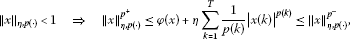(2.10)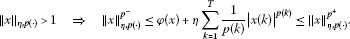(2.11)

## 3 Ground state solutions

We begin by a result which states that the energy functional $\mathcal{I}$ has a minimum point in X provided that the potential of the nonlinearity f lies asymptotically on the left of the first eigenvalue like constant

${\lambda }_{1}:=inf\left\{\frac{{\sum }_{k=1}^{T+1}\frac{{|\mathrm{\Delta }x\left(k-1\right)|}^{p\left(k-1\right)}}{p\left(k-1\right)}}{{\sum }_{k=1}^{T}\frac{{|x\left(k\right)|}^{p\left(k\right)}}{p\left(k\right)}}:x\in X\setminus \left\{0\right\},\left(x\left(0\right),x\left(T+1\right)\right)\in D\left(j\right)\right\}.$
(3.1)

Theorem 3.1 If

$\underset{|t|\to \mathrm{\infty }}{lim sup}\frac{p\left(k\right)F\left(k,t\right)}{{|t|}^{p\left(k\right)}}<{\lambda }_{1},\phantom{\rule{1em}{0ex}}\left(\mathrm{\forall }\right)k\in \left[1,T\right],$
(3.2)

then problem (1.1), (1.2) has at least one solution which minimizes $\mathcal{I}$ on X.

Proof By the continuity of Φ and the lower semicontinuity of ψ, we have that the functional $\mathcal{I}$ is sequentially l.s.c. on X. It remains to prove that $\mathcal{I}$ is coercive on X. Then, by the direct method in calculus of variations, $\mathcal{I}$ is bounded from below and attains its infimum at some $x\in X$, which, by virtue of (, Proposition 1.1) and Proposition 2.1, is a solution of problem (1.1), (1.2).

From (3.2) there are constants $\sigma >0$ and $\rho >0$ such that

If ${\lambda }_{1}>0$, we may assume that $\sigma <{\lambda }_{1}$. On the other hand, by the continuity of F, there is a constant ${M}_{\rho }>0$ such that

Hence, we infer

$F\left(k,t\right)\le {M}_{\rho }+\frac{|{\lambda }_{1}-\sigma |}{p\left(k\right)}{\rho }^{p\left(k\right)}+\frac{{\lambda }_{1}-\sigma }{p\left(k\right)}{|t|}^{p\left(k\right)},\phantom{\rule{1em}{0ex}}\left(\mathrm{\forall }\right)k\in \left[1,T\right],\left(\mathrm{\forall }\right)t\in \mathbb{R}.$

To prove the coercivity of $\mathcal{I}$, from the above inequality, we obtain

$\begin{array}{rcl}\mathcal{I}\left(x\right)& \ge & \phi \left(x\right)-{M}_{\rho }T-|{\lambda }_{1}-\sigma |\sum _{k=1}^{T}\frac{1}{p\left(k\right)}{\rho }^{p\left(k\right)}-\left({\lambda }_{1}-\sigma \right)\sum _{k=1}^{T}\frac{1}{p\left(k\right)}{|x\left(k\right)|}^{p\left(k\right)}+J\left(x\right)\\ \ge & \phi \left(x\right)-{M}_{\rho }T-\frac{|{\lambda }_{1}-\sigma |}{{p}^{-}}\left({\rho }^{{p}^{-}}+{\rho }^{{p}^{+}}\right)T-\left({\lambda }_{1}-\sigma \right)\sum _{k=1}^{T}\frac{1}{p\left(k\right)}{|x\left(k\right)|}^{p\left(k\right)}+J\left(x\right)\\ =& \phi \left(x\right)-\left({\lambda }_{1}-\sigma \right)\sum _{k=1}^{T}\frac{1}{p\left(k\right)}{|x\left(k\right)|}^{p\left(k\right)}-{C}_{1}+J\left(x\right),\phantom{\rule{1em}{0ex}}\left(\mathrm{\forall }\right)x\in X,\end{array}$

where ${C}_{1}={M}_{\rho }T+\frac{|{\lambda }_{1}-\sigma |}{{p}^{-}}\left({\rho }^{{p}^{-}}+{\rho }^{{p}^{+}}\right)T$.

If ${\lambda }_{1}=0$, using (2.11) from Remark 2.2, we have

$\begin{array}{rcl}\mathcal{I}\left(x\right)& \ge & \phi \left(x\right)+\sigma \sum _{k=1}^{T}\frac{1}{p\left(k\right)}{|x\left(k\right)|}^{p\left(k\right)}-{C}_{1}+J\left(x\right)\\ \ge & {\parallel x\parallel }_{\sigma ,p\left(\cdot \right)}^{{p}^{-}}-{C}_{1}+J\left(x\right),\phantom{\rule{1em}{0ex}}\left(\mathrm{\forall }\right)x\in X,{\parallel x\parallel }_{\sigma ,p\left(\cdot \right)}>1.\end{array}$
(3.3)

In the case ${\lambda }_{1}>0$, by virtue of (2.11) and (3.1), for ${\parallel x\parallel }_{{\lambda }_{1},p\left(\cdot \right)}>1$, one obtains

${\parallel x\parallel }_{{\lambda }_{1},p\left(\cdot \right)}^{{p}^{-}}\le \phi \left(x\right)+{\lambda }_{1}\sum _{k=1}^{T}\frac{1}{p\left(k\right)}{|x\left(k\right)|}^{p\left(k\right)}\le 2\phi \left(x\right),$
(3.4)

which, using again (3.1), implies

$\begin{array}{rcl}\mathcal{I}\left(x\right)& \ge & \phi \left(x\right)+\left(\sigma -{\lambda }_{1}\right)\frac{{\sum }_{k=1}^{T+1}\frac{1}{p\left(k-1\right)}{|\mathrm{\Delta }x\left(k-1\right)|}^{p\left(k-1\right)}}{{\lambda }_{1}}-{C}_{1}+J\left(x\right)\\ =& \frac{\sigma }{{\lambda }_{1}}\phi \left(x\right)-{C}_{1}+J\left(x\right)\ge \frac{\sigma }{2{\lambda }_{1}}{\parallel x\parallel }_{{\lambda }_{1},p\left(\cdot \right)}^{{p}^{-}}-{C}_{1}+J\left(x\right),\phantom{\rule{1em}{0ex}}\left(\mathrm{\forall }\right)x\in D\left(J\right).\end{array}$
(3.5)

In both cases, by virtue of (3.3) and (3.5), there exist constants $\eta ,{C}_{2}>0$ such that

$\mathcal{I}\left(x\right)\ge {C}_{2}{\parallel x\parallel }_{\eta ,p\left(\cdot \right)}^{{p}^{-}}-{C}_{1}+J\left(x\right),\phantom{\rule{1em}{0ex}}\left(\mathrm{\forall }\right)x\in D\left(J\right),{\parallel x\parallel }_{\eta ,p\left(\cdot \right)}>1.$

On the other hand, as j is convex and l.s.c., it is bounded from below by an affine functional. Therefore, on account of (2.3), there are positive constants ${k}_{1}$, ${k}_{2}$, ${k}_{3}$ such that

$\begin{array}{rcl}\mathcal{I}\left(x\right)& \ge & {C}_{2}{\parallel x\parallel }_{\eta ,p\left(\cdot \right)}^{{p}^{-}}-{C}_{1}-{k}_{1}|x\left(0\right)|-{k}_{2}|x\left(T+1\right)|-{k}_{3}\\ \ge & {C}_{2}{\parallel x\parallel }_{\eta ,p\left(\cdot \right)}^{{p}^{-}}-{C}_{3}{\parallel x\parallel }_{\mathrm{\infty }}-{C}_{4},\phantom{\rule{1em}{0ex}}\left(\mathrm{\forall }\right)x\in D\left(J\right)\end{array}$

with ${C}_{3}={k}_{1}+{k}_{2}$ and ${C}_{4}={C}_{1}+{k}_{3}$. Since any norm on X is equivalent to ${\parallel \cdot \parallel }_{\eta ,p\left(\cdot \right)}$, there exists ${C}_{5}>0$ such that

$\mathcal{I}\left(x\right)\ge {C}_{2}{\parallel x\parallel }_{\eta ,p\left(\cdot \right)}^{{p}^{-}}-{C}_{5}{\parallel x\parallel }_{\eta ,p\left(\cdot \right)}-{C}_{4}.$

Consequently,

meaning that $\mathcal{I}$ is coercive on $\left(X,{\parallel \cdot \parallel }_{\eta ,p\left(\cdot \right)}\right)$ and the proof is complete. □

In order to give an application of Theorem 3.1, we consider the problem

$\left\{\begin{array}{c}-{\mathrm{\Delta }}_{p\left(k-1\right)}x\left(k-1\right)=\lambda g\left(k,x\left(k\right)\right),\phantom{\rule{1em}{0ex}}\left(\mathrm{\forall }\right)k\in \left[1,T\right],\hfill \\ \left({h}_{p\left(0\right)}\left(\mathrm{\Delta }x\left(0\right)\right),-{h}_{p\left(T\right)}\left(\mathrm{\Delta }x\left(T\right)\right)\right)\in \partial j\left(x\left(0\right),x\left(T+1\right)\right),\hfill \end{array}$
(3.6)

where $g:\left[1,T\right]×\mathbb{R}\to \mathbb{R}$ is a continuous function and λ is a positive parameter.

Corollary 3.2 Assume that ${\lambda }_{1}>0$ and g satisfies the growth condition

$|g\left(k,t\right)|\le a{|t|}^{q\left(k\right)}+b,\phantom{\rule{1em}{0ex}}\left(\mathrm{\forall }\right)k\in \left[1,T\right],\left(\mathrm{\forall }\right)t\in \mathbb{R},$
(3.7)

where $a>0$, $b\in {\mathbb{R}}_{+}$ are constants and $q:\left[1,T\right]\to \left[0,\mathrm{\infty }\right)$. The following hold true:

(i) if $\underline{p}>\overline{q}+1$, then problem (3.6) has a solution for any $\lambda >0$;

(ii) if $\underline{p}=\overline{q}+1$, then there is some ${\lambda }^{\star }>0$ such that for any $\lambda \in \left(0,{\lambda }^{\star }\right)$, problem (3.6) has a solution.

Proof We apply Theorem 3.1 with $f\left(k,t\right)=\lambda g\left(k,t\right)$ for all $k\in \left[1,T\right]$ and $t\in \mathbb{R}$. From (3.7), we obtain

$\begin{array}{rcl}|F\left(k,t\right)|& =& |{\int }_{0}^{t}f\left(k,\tau \right)\phantom{\rule{0.2em}{0ex}}d\tau |\le \lambda {\int }_{0}^{|t|}\left(a{\tau }^{q\left(k\right)}+b\right)\phantom{\rule{0.2em}{0ex}}d\tau \\ =& \lambda a\frac{{|t|}^{q\left(k\right)+1}}{q\left(k\right)+1}+\lambda |t|b\le \frac{\lambda a}{\underline{q}+1}{|t|}^{q\left(k\right)+1}+\lambda |t|b,\phantom{\rule{1em}{0ex}}\left(\mathrm{\forall }\right)k\in \left[1,T\right],\left(\mathrm{\forall }\right)t\in \mathbb{R}.\end{array}$

Thus, we deduce

$\frac{p\left(k\right)F\left(k,t\right)}{{|t|}^{p\left(k\right)}}\le \frac{\overline{p}\lambda a}{\underline{q}+1}\frac{{|t|}^{q\left(k\right)+1}}{{|t|}^{p\left(k\right)}}+\frac{\overline{p}\lambda b|t|}{{|t|}^{p\left(k\right)}}\le \frac{\overline{p}\lambda a}{\underline{q}+1}\frac{{|t|}^{\overline{q}+1}}{{|t|}^{\underline{p}}}+\frac{\overline{p}\lambda b|t|}{{|t|}^{\underline{p}}}$

for all $k\in \left[1,T\right]$ and $t\in \mathbb{R}$ with $|t|>1$. So, if $\underline{p}>\overline{q}+1$, then

$\underset{|t|\to \mathrm{\infty }}{lim sup}\frac{p\left(k\right)F\left(k,t\right)}{{|t|}^{p\left(k\right)}}\le 0<{\lambda }_{1},\phantom{\rule{1em}{0ex}}\left(\mathrm{\forall }\right)k\in \left[1,T\right].$

Also, if $\underline{p}=\overline{q}+1$, setting

${\lambda }^{\star }=\frac{{\lambda }_{1}\left(\underline{q}+1\right)}{a\overline{p}},$
(3.8)

it is easy to see that condition (3.2) is fulfilled for any $\lambda \in \left(0,{\lambda }^{\star }\right)$. □

Remark 3.3

(i) Note that a valid ${\lambda }^{\star }$ in Corollary 3.2(ii) is given by formula (3.8).

(ii) Theorem 5 in  is an immediate consequence of Corollary 3.2 with $j={I}_{K}$, $K=\left\{\left(0,0\right)\right\}$.

## 4 Mountain pass type solutions

In this section, we deal with the existence of nontrivial solutions for the equation

$-{\mathrm{\Delta }}_{p\left(k-1\right)}x\left(k-1\right)+r\left(k\right){h}_{p\left(k\right)}\left(x\left(k\right)\right)=f\left(k,x\left(k\right)\right),\phantom{\rule{1em}{0ex}}\left(\mathrm{\forall }\right)k\in \left[1,T\right],$
(4.1)

associated with the potential boundary condition (1.2). Here, f and j are as in the case of the previous problem (1.1), (1.2) and $r:\left[1,T\right]\to \left[0,\mathrm{\infty }\right)$ is a given function. The main tool in obtaining such a result will be the Mountain Pass Theorem .

To treat problem (4.1), (1.2), instead of φ, there will be ${\phi }_{r}:X\to \mathbb{R}$ defined by

${\phi }_{r}\left(x\right)=\sum _{k=1}^{T+1}\frac{1}{p\left(k-1\right)}{|\mathrm{\Delta }x\left(k-1\right)|}^{p\left(k-1\right)}+\sum _{k=1}^{T}\frac{r\left(k\right)}{p\left(k\right)}{|x\left(k\right)|}^{p\left(k\right)},\phantom{\rule{1em}{0ex}}\left(\mathrm{\forall }\right)x\in X,$
(4.2)

which is convex, of class ${C}^{1}$ on X, and its derivative is given by

$\begin{array}{rcl}〈{\phi }_{r}^{\prime }\left(x\right),y〉& =& \sum _{k=1}^{T+1}{h}_{p\left(k-1\right)}\left(\mathrm{\Delta }x\left(k-1\right)\right)\mathrm{\Delta }y\left(k-1\right)\\ +\sum _{k=1}^{T}r\left(k\right){h}_{p\left(k\right)}\left(x\left(k\right)\right)y\left(k\right),\phantom{\rule{1em}{0ex}}\left(\mathrm{\forall }\right)x,y\in X.\end{array}$
(4.3)

Then, setting ${\psi }_{r}={\phi }_{r}+J$, we define

${\mathcal{I}}_{r}={\psi }_{r}+\mathrm{\Phi },$
(4.4)

with J given by (2.3) and Φ in (2.5).

By means of ${\lambda }_{1}$ in (3.1), we define the constants

$\underline{\eta }:={\lambda }_{1}+\underline{r}\phantom{\rule{1em}{0ex}}\text{and}\phantom{\rule{1em}{0ex}}\overline{\eta }:={\lambda }_{1}+\overline{r}.$
(4.5)

Lemma 4.1 If $\underline{\eta }>0$ and there exist constants $\theta >{p}^{+}$ and ${C}_{1},\rho >0$ such that

${j}^{\prime }\left(z;z\right)\le \theta j\left(z\right)+{C}_{1},\phantom{\rule{1em}{0ex}}\left(\mathrm{\forall }\right)z\in D\left(j\right)$
(4.6)

and

$\theta F\left(k,t\right)\le tf\left(k,t\right),\phantom{\rule{1em}{0ex}}\left(\mathrm{\forall }\right)k\in \left[1,T\right],\left(\mathrm{\forall }\right)t\in \mathbb{R}\mathit{\text{with}}|t|>\rho ,$
(4.7)

then the functional ${\mathcal{I}}_{r}$ defined in (4.4) satisfies the Palais-Smale condition ((PS) condition for short) on $\left(X,{\parallel \cdot \parallel }_{\underline{\eta },p\left(\cdot \right)}\right)$, i.e., every sequence $\left\{{x}_{n}\right\}\subset X$ for which ${\mathcal{I}}_{r}\left({x}_{n}\right)\to c\in \mathbb{R}$ and

$〈{\mathrm{\Phi }}^{\prime }\left({x}_{n}\right),y-{x}_{n}〉+{\psi }_{r}\left(y\right)-{\psi }_{r}\left({x}_{n}\right)\ge -{\epsilon }_{n}{\parallel y-{x}_{n}\parallel }_{\underline{\eta },p\left(\cdot \right)},\phantom{\rule{1em}{0ex}}\left(\mathrm{\forall }\right)y\in X,$
(4.8)

where ${\epsilon }_{n}\to 0$, possesses a convergent subsequence.

Proof Let $\left\{{x}_{n}\right\}\subset X$ be a sequence for which ${\mathcal{I}}_{r}\left({x}_{n}\right)\to c\in \mathbb{R}$ and (4.8) holds true with ${\epsilon }_{n}\to 0$. Since X is finite dimensional, it is sufficient to prove that $\left\{{x}_{n}\right\}$ is bounded. In order to show this, we may assume that $\left\{{x}_{n}\right\}\subset D\left({\mathcal{I}}_{r}\right)=D\left(J\right)$ and ${\parallel {x}_{n}\parallel }_{\underline{\eta },p\left(\cdot \right)}>1$ for all $n\in \mathbb{N}$. By virtue of (2.11), (3.1) and (4.5), we get

$\begin{array}{rcl}{\parallel {x}_{n}\parallel }_{\underline{\eta },p\left(\cdot \right)}^{{p}^{-}}& \le & \sum _{k=1}^{T+1}\frac{{|\mathrm{\Delta }{x}_{n}\left(k-1\right)|}^{p\left(k-1\right)}}{p\left(k-1\right)}+\left({\lambda }_{1}+\underline{r}\right)\sum _{k=1}^{T}\frac{1}{p\left(k\right)}{|{x}_{n}\left(k\right)|}^{p\left(k\right)}\\ \le & \frac{2}{{p}^{-}}\left(\sum _{k=1}^{T+1}{|\mathrm{\Delta }{x}_{n}\left(k-1\right)|}^{p\left(k-1\right)}+\sum _{k=1}^{T}r\left(k\right){|{x}_{n}\left(k\right)|}^{p\left(k\right)}\right).\end{array}$
(4.9)

From (2.3) and (4.6), it follows

$J\left(y\right)-\frac{1}{\theta }{J}^{\prime }\left(y;y\right)\ge -{C}_{2},\phantom{\rule{1em}{0ex}}\left(\mathrm{\forall }\right)y\in D\left(J\right)$
(4.10)

with ${C}_{2}={C}_{1}/\theta$. Using (4.7) we deduce that, for all $n\in \mathbb{N}$, it holds

$\begin{array}{rcl}-\mathrm{\Phi }\left({x}_{n}\right)+\frac{1}{\theta }〈{\mathrm{\Phi }}^{\prime }\left({x}_{n}\right),{x}_{n}〉& =& \frac{1}{\theta }\sum _{k=1}^{T}\left[\theta F\left(k,{x}_{n}\left(k\right)\right)-{x}_{n}\left(k\right)f\left(k,{x}_{n}\left(k\right)\right)\right]\\ \le & \frac{1}{\theta }\sum _{|{x}_{n}\left(k\right)|\le \rho }\left[\theta F\left(k,{x}_{n}\left(k\right)\right)-{x}_{n}\left(k\right)f\left(k,{x}_{n}\left(k\right)\right)\right]\\ \le & \frac{1}{\theta }\sum _{k=1}^{T}\underset{|x|\le \rho }{max}|\theta F\left(k,x\right)-xf\left(k,x\right)|=:{C}_{3}.\end{array}$
(4.11)

Clearly, there is a constant ${C}_{4}>0$, such that

$|{\mathcal{I}}_{r}\left({x}_{n}\right)|\le {C}_{4},\phantom{\rule{1em}{0ex}}\left(\mathrm{\forall }\right)n\in \mathbb{N}.$
(4.12)

Further, setting $y={x}_{n}+s{x}_{n}$ in (4.8), dividing by $s>0$ and then letting $s\to {0}^{+}$, we obtain

$〈{\mathrm{\Phi }}^{\prime }\left({x}_{n}\right),{x}_{n}〉+〈{\phi }_{r}^{\prime }\left({x}_{n}\right),{x}_{n}〉+{J}^{\prime }\left({x}_{n};{x}_{n}\right)\ge -{\epsilon }_{n}{\parallel {x}_{n}\parallel }_{\underline{\eta },p\left(\cdot \right)},\phantom{\rule{1em}{0ex}}\left(\mathrm{\forall }\right)n\in \mathbb{N}.$
(4.13)

Using (4.12) and (4.13), we deduce that

$\begin{array}{rcl}{C}_{4}+\frac{{\epsilon }_{n}}{\theta }{\parallel {x}_{n}\parallel }_{\underline{\eta },p\left(\cdot \right)}& \ge & \mathrm{\Phi }\left({x}_{n}\right)+{\phi }_{r}\left({x}_{n}\right)+J\left({x}_{n}\right)+\frac{{\epsilon }_{n}}{\theta }{\parallel {x}_{n}\parallel }_{\underline{\eta },p\left(\cdot \right)}\\ \ge & \mathrm{\Phi }\left({x}_{n}\right)-\frac{1}{\theta }〈{\mathrm{\Phi }}^{\prime }\left({x}_{n}\right),{x}_{n}〉+{\phi }_{r}\left({x}_{n}\right)-\frac{1}{\theta }〈{\phi }_{r}^{\prime }\left({x}_{n}\right),{x}_{n}〉+J\left({x}_{n}\right)-\frac{1}{\theta }{J}^{\prime }\left({x}_{n};{x}_{n}\right)\end{array}$

and by virtue of (4.10), (4.11), (4.3) and (4.9), we have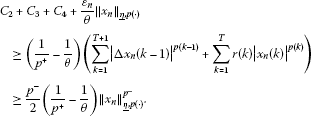Since $\theta >{p}^{+}$, we infer that $\left\{{x}_{n}\right\}$ is bounded and the proof is complete. □

Now, we can state the following result of Ambrosetti-Rabinowitz type .

Theorem 4.2 Assume that $\underline{\eta }>0$ and, in addition,

(i) $\left(0,0\right)\in \partial j\left(0,0\right)$;

(ii) ${lim sup}_{|t|\to 0}\frac{p\left(k\right)F\left(k,t\right)}{{|t|}^{p\left(k\right)}}<\underline{\eta }$, $\left(\mathrm{\forall }\right)k\in \left[1,T\right]$;

(iii) there are constants $\theta >{p}^{+}$ and ${C}_{1},\rho >0$ such that (4.6) holds true and

$0<\theta F\left(k,t\right)\le tf\left(k,t\right),\phantom{\rule{1em}{0ex}}\left(\mathrm{\forall }\right)k\in \left[1,T\right],\left(\mathrm{\forall }\right)t\in \mathbb{R}\mathit{\text{with}}|t|>\rho .$
(4.14)

Then, problem (4.1), (1.2) has a nontrivial solution.

Proof Without loss of generality, we may assume that

$j\left(0,0\right)=0,$
(4.15)

which implies that ${\mathcal{I}}_{r}\left(0\right)=0$. From (i), (2.3) and (4.15), we have

$J\left(x\right)\ge J\left(0\right)=0,\phantom{\rule{1em}{0ex}}\left(\mathrm{\forall }\right)x\in D\left(J\right).$
(4.16)

From Lemma 4.1 and (iii), the functional ${\mathcal{I}}_{r}$ satisfies the (PS) condition on $\left(X,{\parallel \cdot \parallel }_{\underline{\eta },p\left(\cdot \right)}\right)$.

Next, we shall prove that ${\mathcal{I}}_{r}$ has a ‘mountain pass’ geometry:

(a) there exist $\alpha ,\mu >0$ such that ${\mathcal{I}}_{r}\left(x\right)\ge \alpha$ if ${\parallel x\parallel }_{\underline{\eta },p\left(\cdot \right)}=\mu$;

(b) ${\mathcal{I}}_{r}\left(e\right)\le 0$ for some $e\in X$ with ${\parallel e\parallel }_{\underline{\eta },p\left(\cdot \right)}>\mu$.

By the equivalence of the norms on X, there is some ${C}_{2}>0$ such that

${\parallel x\parallel }_{\mathrm{\infty }}\le {C}_{2}{\parallel x\parallel }_{\underline{\eta },p\left(\cdot \right)},\phantom{\rule{1em}{0ex}}\left(\mathrm{\forall }\right)x\in X.$
(4.17)

Using (ii) we can find constants $\sigma \in \left(0,\underline{\eta }\right)$ and $\mu \in \left(0,1\right)$ such that

(4.18)

Let $x\in X$, with ${\parallel x\parallel }_{\underline{\eta },p\left(\cdot \right)}\le \mu$, be arbitrarily chosen. From (4.17) and (4.18), we have

$F\left(k,x\left(k\right)\right)\le \frac{\underline{\eta }-\sigma }{p\left(k\right)}{|x\left(k\right)|}^{p\left(k\right)},\phantom{\rule{1em}{0ex}}\left(\mathrm{\forall }\right)k\in \left[1,T\right],$

which implies

$-\mathrm{\Phi }\left(x\right)\le \left(\underline{\eta }-\sigma \right)\sum _{k=1}^{T}\frac{1}{p\left(k\right)}{|x\left(k\right)|}^{p\left(k\right)}.$

Now, using (2.10) and (3.1), we get

$\begin{array}{rcl}{\parallel x\parallel }_{\underline{\eta },p\left(\cdot \right)}^{{p}^{+}}& \le & \sum _{k=1}^{T+1}\frac{{|\mathrm{\Delta }x\left(k-1\right)|}^{p\left(k-1\right)}}{p\left(k-1\right)}\\ +\left({\lambda }_{1}+\underline{r}\right)\sum _{k=1}^{T}\frac{1}{p\left(k\right)}{|x\left(k\right)|}^{p\left(k\right)}\le 2{\phi }_{r}\left(x\right).\end{array}$
(4.19)

By virtue of (3.1), (4.5), (4.2) and (4.19), we deduce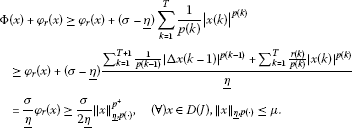On account of (4.16), we infer that

(4.20)

with $\frac{\sigma {\mu }^{{p}^{+}}}{2\underline{\eta }}=:\alpha >0$, and condition (a) is fulfilled.

Our next task is to prove that ${\mathcal{I}}_{r}$ satisfies condition (b). To this end, let us first observe that, by virtue of (4.14), there exist ${a}_{1},{a}_{2}>0$ such that

$F\left(k,t\right)\ge {a}_{1}{|t|}^{\theta }-{a}_{2},\phantom{\rule{1em}{0ex}}\left(\mathrm{\forall }\right)k\in \left[1,T\right],\left(\mathrm{\forall }\right)t\in \mathbb{R}.$
(4.21)

Let ${x}_{0}\in X\setminus \left\{0\right\}$ be such that ${x}_{0}\left(0\right)={x}_{0}\left(T+1\right)=0$ and ${\parallel {x}_{0}\parallel }_{\overline{\eta },p\left(\cdot \right)}>1$. Using (2.11) and (4.5), one obtains

${\parallel {x}_{0}\parallel }_{\overline{\eta },p\left(\cdot \right)}^{{p}^{+}}\ge \sum _{k=1}^{T+1}\frac{{|\mathrm{\Delta }{x}_{0}\left(k-1\right)|}^{p\left(k-1\right)}}{p\left(k-1\right)}+\left({\lambda }_{1}+\overline{r}\right)\sum _{k=1}^{T}\frac{1}{p\left(k\right)}{|{x}_{0}\left(k\right)|}^{p\left(k\right)}\ge {\phi }_{r}\left({x}_{0}\right).$
(4.22)

From (4.15), we have that

$J\left(s{x}_{0}\right)=0,\phantom{\rule{1em}{0ex}}\left(\mathrm{\forall }\right)s\in \mathbb{R},$

which, together with (4.21) and (4.22) for any $s\ge 1$, gives

${\mathcal{I}}_{r}\left(s{x}_{0}\right)\le {s}^{{p}^{+}}{\phi }_{r}\left({x}_{0}\right)+\mathrm{\Phi }\left(s{x}_{0}\right)\le {s}^{{p}^{+}}{\parallel {x}_{0}\parallel }_{\overline{\eta },p\left(\cdot \right)}^{{p}^{+}}-{s}^{\theta }{a}_{1}\sum _{k=1}^{T}{|{x}_{0}\left(k\right)|}^{\theta }+{a}_{2}T\to -\mathrm{\infty },$

as $s\to +\mathrm{\infty }$ because $\theta >{p}^{+}$. Hence, we can choose ${s}_{0}$ large enough to satisfy ${\mathcal{I}}_{r}\left({s}_{0}{x}_{0}\right)\le 0$ and ${\parallel {s}_{0}{x}_{0}\parallel }_{\underline{\eta },p\left(\cdot \right)}>\mu$, with μ entering in (4.20). This means that condition (b) is satisfied with $e={s}_{0}{x}_{0}$. □

## 5 An application

In this section, we show how Theorem 4.2 can be applied to derive the existence of nontrivial solutions for equation (4.1) associated with some concrete boundary conditions.

Let $g:\mathbb{R}×\mathbb{R}\to \mathbb{R}$ be a convex and Gâteaux differentiable function with $dg\left(0,0\right)=\left(0,0\right)$, where dg denotes the differential of g. Also, given a nonempty closed convex cone $K\subset \mathbb{R}×\mathbb{R}$, we denote by ${N}_{K}\left(z\right)$ the normal cone to K at $z\in K$, i.e.,

${N}_{K}\left(z\right)=\left\{\zeta \in \mathbb{R}×\mathbb{R}:\left(\zeta |\xi -z\right)\le 0,\left(\mathrm{\forall }\right)\xi \in K\right\},\phantom{\rule{1em}{0ex}}\left(\mathrm{\forall }\right)z\in K.$

The equation (4.1) is considered to be associated with the boundary conditions

$\begin{array}{r}\left(x\left(0\right),x\left(T+1\right)\right)\in K,\\ \left({h}_{p\left(0\right)}\left(\mathrm{\Delta }x\left(0\right)\right),-{h}_{p\left(T\right)}\left(\mathrm{\Delta }x\left(T\right)\right)\right)-dg\left(x\left(0\right),x\left(T+1\right)\right)\in {N}_{K}\left(x\left(0\right),x\left(T+1\right)\right).\end{array}$
(5.1)

We set

${\underline{\eta }}_{K}:=\underline{r}+inf\left\{\frac{{\sum }_{k=1}^{T+1}\frac{1}{p\left(k-1\right)}{|\mathrm{\Delta }x\left(k-1\right)|}^{p\left(k-1\right)}}{{\sum }_{k=1}^{T}\frac{1}{p\left(k\right)}{|x\left(k\right)|}^{p\left(k\right)}}:x\in X\setminus \left\{0\right\},\left(x\left(0\right),x\left(T+1\right)\right)\in K\right\}.$

Theorem 5.1 If $f:\left[1,T\right]×\mathbb{R}\to \mathbb{R}$ is continuous, ${\underline{\eta }}_{K}>0$ and, in addition, we assume that

(i) ${lim sup}_{|t|\to 0}\frac{p\left(k\right)F\left(k,t\right)}{{|t|}^{p\left(k\right)}}<{\underline{\eta }}_{K}$, $\left(\mathrm{\forall }\right)k\in \left[1,T\right]$;

(ii) there are constants $\theta >{p}^{+}$ and ${C}_{1},\rho >0$ such that (4.14) holds true and

$〈dg\left(z\right),z〉\le \theta g\left(z\right)+{C}_{1},\phantom{\rule{1em}{0ex}}\left(\mathrm{\forall }\right)z\in K,$
(5.2)

then problem (4.1), (5.1) has a nontrivial solution.

Proof Since ${N}_{K}\left(z\right)=\partial {I}_{K}\left(z\right)$ for all $z\in K$, Theorem 4.2 applies with $j\left(z\right)=g\left(z\right)+{I}_{K}\left(z\right)$, $\left(\mathrm{\forall }\right)z\in \mathbb{R}×\mathbb{R}$. □

Remark 5.2 Conditions (5.1) allow various possible choices of g and K, which, among others, recover classical boundary conditions. For instance, if $g=0$, then the homogeneous boundary conditions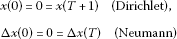are obtained by choosing $K=\left\{\left(0,0\right)\right\}$, respectively $K=\mathbb{R}×\mathbb{R}$. If, in addition, p is T-periodic, then taking $K=\left\{\left(x,x\right),x\in \mathbb{R}\right\}$ and $K=\left\{\left(x,-x\right),x\in \mathbb{R}\right\}$, we get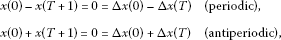respectively. If the T-periodicity condition is not assumed, then we only have

${h}_{p\left(0\right)}\left(\mathrm{\Delta }x\left(0\right)\right)={h}_{p\left(T\right)}\left(\mathrm{\Delta }x\left(T\right)\right)\phantom{\rule{1em}{0ex}}\text{and}\phantom{\rule{1em}{0ex}}{h}_{p\left(0\right)}\left(\mathrm{\Delta }x\left(0\right)\right)=-{h}_{p\left(T\right)}\left(\mathrm{\Delta }x\left(T\right)\right)$

instead of $\mathrm{\Delta }x\left(0\right)=\mathrm{\Delta }x\left(T\right)$ and $\mathrm{\Delta }x\left(0\right)=-\mathrm{\Delta }x\left(T\right)$, respectively. As $g=0$, in these four cases, condition (5.2) is automatically satisfied with any $\theta \in \mathbb{R}$ and ${C}_{1}=0$.

Also, if $\alpha ,\beta >0$ are given, then with g defined by

$g\left(z\right):=\frac{1}{p\left(0\right)}\frac{{|{z}_{1}|}^{p\left(0\right)}}{{\alpha }^{p\left(0\right)-1}}+\frac{1}{p\left(T\right)}\frac{{|{z}_{2}|}^{p\left(T\right)}}{{\beta }^{p\left(T\right)-1}},\phantom{\rule{1em}{0ex}}\left(\mathrm{\forall }\right)z=\left({z}_{1},{z}_{2}\right)\in \mathbb{R}×\mathbb{R}$

and $K=\mathbb{R}×\mathbb{R}$, we deduce the Sturm-Liouville type boundary conditions

$x\left(0\right)-\alpha \mathrm{\Delta }x\left(0\right)=0,\phantom{\rule{2em}{0ex}}x\left(T+1\right)+\beta \mathrm{\Delta }x\left(T\right)=0.$

In this case, (5.2) is fulfilled with any $\theta \ge min\left\{p\left(0\right),p\left(T\right)\right\}$ and ${C}_{1}=0$.

Therefore, sufficient conditions ensuring the existence of nontrivial solutions of (4.1) subjected to one of the above boundary conditions can be easily stated by means of Theorem 5.1.

Remark 5.3 It is worth pointing out that in the cases of Dirichlet and antiperiodic boundary conditions, $\underline{r}$ is allowed to be =0, and hence, r may be ≥0 on $\left[1,T\right]$; while in the Neumann, periodic and Sturm-Liouville cases, $\underline{r}$ must be >0, meaning $r>0$ on $\left[1,T\right]$.

## References

1. Gasinski L, Papageorgiou NS: Nonlinear second-order multivalued boundary value problems. Proc. Indian Acad. Sci. Math. Sci. 2003, 113: 293-319. 10.1007/BF02829608

2. Jebelean P: Variational methods for ordinary p -Laplacian systems with potential boundary conditions. Adv. Differ. Equ. 2008, 13(3-4):273-322.

3. Agarwal RP, Perera K, O’Regan D: Multiple positive solutions of singular discrete p -Laplacian problems via variational methods. Adv. Differ. Equ. 2005, 2005(2):93-99.

4. Cabada A, Iannizzotto A, Tersian S: Multiple solutions for discrete boundary value problems. J. Math. Anal. Appl. 2009, 356: 418-428. 10.1016/j.jmaa.2009.02.038

5. Candito P, D’Agui G: Three solutions for a discrete nonlinear Neumann problem involving p -Laplacian. Adv. Differ. Equ. 2010., 2010: Article ID 862016

6. Tian Y, Ge W: The existence of solutions for a second-order discrete Neumann problem with a p -Laplacian. J. Appl. Math. Comput. 2008, 26: 333-340. 10.1007/s12190-007-0012-5

7. He T, Chen W: Periodic solutions of second order discrete convex systems involving the p -Laplacian. Appl. Math. Comput. 2008, 206: 124-132. 10.1016/j.amc.2008.08.037

8. Jebelean P, Şerban C: Ground state periodic solutions for difference equations with discrete p -Laplacian. Appl. Math. Comput. 2011, 217: 9820-9827. 10.1016/j.amc.2011.04.076

9. Mawhin J: Periodic solutions of second order nonlinear difference systems with ϕ -Laplacian: a variational approach. Nonlinear Anal. 2012, 75(12):4672-4687. 10.1016/j.na.2011.11.018

10. Bereanu C, Jebelean P, Şerban C:Periodic and Neumann problems for discrete $p\left(\cdot \right)$-Laplacian. J. Math. Anal. Appl. 2012. doi:10.1016/j.jmaa.2012.09.047

11. Galewski M, Gła̧b S: On the discrete boundary value problem for anisotropic equation. J. Math. Anal. Appl. 2012, 386: 956-965. 10.1016/j.jmaa.2011.08.053

12. Galewski, M, Gła̧b, S: Positive solutions for anisotropic discrete BVP. arXiv:1203.4504v1

13. Guiro A, Nyanquini I, Ouaro S:On the solvability of discrete nonlinear Neumann problems involving the $p\left(x\right)$-Laplacian. Adv. Differ. Equ. 2011., 2011: Article ID 32

14. Koné B, Ouaro S: Weak solutions for anisotropic discrete boundary value problems. J. Differ. Equ. Appl. 2010, 16(2):1-11.

15. Mashiyev R, Yucedag Z, Ogras S:Existence and multiplicity of solutions for a Dirichlet problem involving the discrete $p\left(x\right)$-Laplacian operator. Electron. J. Qual. Theory Differ. Equ. 2011, 67: 1-10.

16. Mihăilescu M, Rădulescu V, Tersian S: Eigenvalue problems for anisotropic discrete boundary value problems. J. Differ. Equ. Appl. 2009, 15(6):557-567. 10.1080/10236190802214977

17. Mihăilescu M, Rădulescu V, Tersian S: Homoclinic solutions of difference equations with variable exponents. Topol. Methods Nonlinear Anal. 2011, 38: 277-289.

18. Jebelean P, Moroşanu G: Ordinary p -Laplacian systems with nonlinear boundary conditions. J. Math. Anal. Appl. 2006, 313: 738-753. 10.1016/j.jmaa.2005.04.022

19. Şerban C: Existence of solutions for discrete p -Laplacian with potential boundary conditions. J. Differ. Equ. Appl. 2012. doi:10.1080/10236198.2012.657631

20. Szulkin A: Minimax principles for lower semicontinuous functions and applications to nonlinear boundary value problems. Ann. Inst. Henri Poincaré, Anal. Non Linéaire 1986, 3(2):77-109.

21. Ambrosetti A, Rabinowitz PH: Dual variational methods in critical point theory and applications. J. Funct. Anal. 1973, 14: 349-381. 10.1016/0022-1236(73)90051-7

## Acknowledgements

Dedicated to Professor Jean Mawhin for his 70th anniversary.

The research of CŞ was supported by the strategic grant POSDRU/CPP107/DMI1.5/S/78421, Project ID 78421 (2010), co-financed by the European Social Fund - Investing in People, within the Sectoral Operational Programme Human Resources Development 2007-2013. Also, the support for CB and PJ from the grant TE-PN-II-RU-TE-2011-3-0157 (CNCS-Romania) is gratefully acknowledged.

## Author information

Authors

### Corresponding author

Correspondence to Cristian Bereanu.

### Competing interests

The authors declare that they have no competing interests.

### Authors’ contributions

The authors declare that the study was realized in collaboration with the same responsibility. All authors read and approved the final manuscript.

## Rights and permissions

Reprints and Permissions

Bereanu, C., Jebelean, P. & Şerban, C. Ground state and mountain pass solutions for discrete $p\left(\cdot \right)$-Laplacian. Bound Value Probl 2012, 104 (2012). https://doi.org/10.1186/1687-2770-2012-104

• Accepted:

• Published:

• DOI: https://doi.org/10.1186/1687-2770-2012-104

### Keywords

• discrete $p\left(\cdot \right)$-Laplacian operator
• variational methods
• critical point
• Palais-Smale condition
• Mountain Pass Theorem